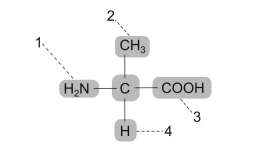# IMAT 2015 Q30 [Amino Acids]

The diagram below shows an amino acid.Which option correctly identifies both the variable group (R group) and the acidic group?

A. variable group = 4; acidic group = 3
B. variable group = 3; acidic group = 1
C. variable group = 2; acidic group = 3
D. variable group = 1; acidic group = 2
E. variable group = 2; acidic group = 4

The answer to this question is choice C.

Let’s label each of the parts of the amino acid according to the diagram given to you in this question:

1. This is the basic amino group. (-NH2)
2. This is the R-group or the side chain that makes the different amino acids, and gives them their unique identity.
3. This is the acidic carboxyl group (-COOH)
4. This is just the hydrogen attached to the central carbon or the alpha carbon.

Thus, with that being said, only choice C is the correct answer.